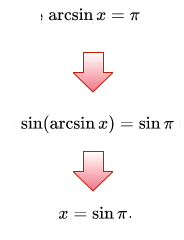# Can We Trust Her?

Geometry Level 1Michaelle asked the classroom to solve $\arcsin x=\pi$ for $x$. After some minutes, she wrote the equation on the blackboard. She solved it as follows:

"Due to the fact that the arcsine of $x$ is defined as the inverse sine function of $x$, we can write $\sin\left(\arcsin x\right)=\sin\pi \Rightarrow x=\sin\pi$. We have that $\sin\pi=0$, so the unique solution to this equation is $x=0$."

Is this reasoning correct?

×

Problem Loading...

Note Loading...

Set Loading...